##### Algebra II For Dummies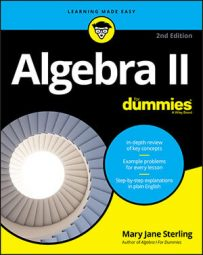Three famous problems confounded mathematicians for centuries: squaring the circle, trisecting the angle, and doubling the cube. These problems are quite accessible nowadays with modern computing and technology. But the ancients only had a compass and straightedge to work with, so these problems were pretty much unsolvable.

While trying to solve the doubling the cube problem, mathematicians discovered conic equations. This situation is somewhat like the accidental discovery of penicillin, where Fleming was researching the flu and found some mold in his petri dish. Like the mathematicians of old, Fleming didn’t discard his discovery, and the rest is history.

But the story starts with doubling the cube. Picture a 1-x-1-x-1-inch cube. Its volume is 1 x 1 x 1 = 1 cubic inch. If you double the length of the sides, you have 2 x 2 x 2 = 8 cubic inches, but that isn’t doubling the volume of the original cube, which is what the ancients wanted. They wanted some a x a x a = 2; they wanted the volume to be doubled.

This problem seems like a simple one! You just need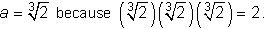But this cube root couldn’t be constructed with compass and straightedge. It was an unsolvable problem, but working on it resulted in the unintentional discovery of conics! How did that come about?

Hippocrates of Chios is credited with constructing some mean proportions to try to solve the doubling problem. From the mean proportions, the equations of conics were derived. A mean proportion, of course, is a proportion in which the means are equal.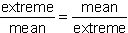For example,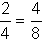is a proportion in which the means are equal. What Hippocrates did was to write the proportions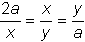and let the ratios in each fraction be equal to r, the ratio he wanted to solve the doubling problem. So if each ratio equals r, then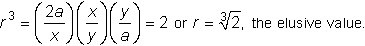But look at the proportions again: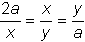If you take the first two ratios,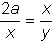and cross multiply, you have x2 = 2ay, the equation of a parabola. Take the last two ratios,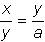and cross multiply. You have ax = y2 another parabola. The first and last ratios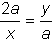have a cross-product of 2a2 = xy, the hyperbola. An unintentional consequence that has impacted mathematics for centuries!